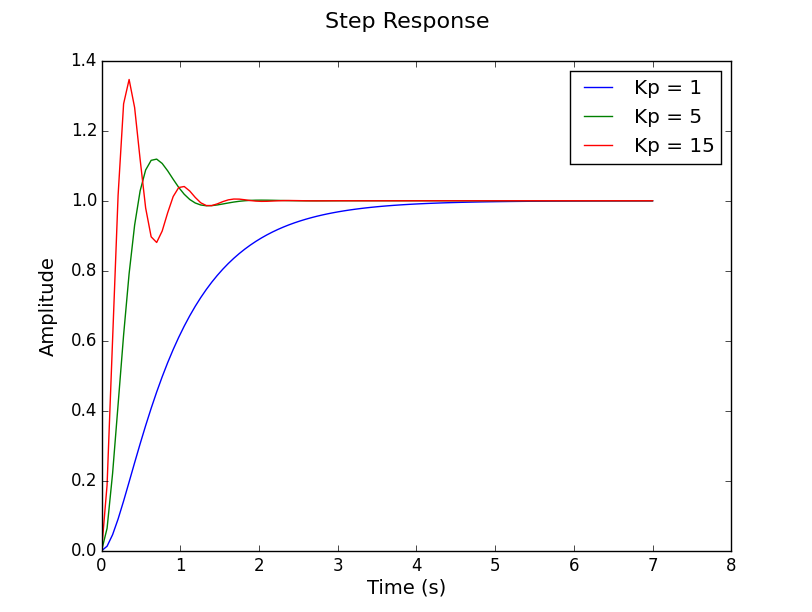203

88

51

19

# ControlSystems.jl

A control systems design toolbox for Julia.

## Installation

To install, in the Julia REPL:

``````Pkg.add("ControlSystems")
``````

## News

### 2019-11-03

• Poles and zeros are "not sorted" as in Julia versions < 1.2, even on newer versions of Julia. This should imply that complex conjugates are kept together.

### 2018-09-01

• LTISystem types are now more generic and can hold matrices/vectors of arbitrary type. Examples (partly pseudo-code): ``` ss(1) ss(1.) ss(1im) ss(ForwardDiff.Dual(1.)) ss(GPUArray()) ss(SparseMatrix()) ``` Similar for `tf,zpk` etc.
• Continuous time systems are simulated with continuous time solvers from `OrdinaryDiffEq.jl`
• Freqresp now returns frequencies in the first dimension.
• Breaking: `lsim(sys, u::Function)` syntax has changed from `u(t,x)` to `u(x,t)` to be consistent with `OrdinaryDiffEq`
• Breaking: `feedback(P,C)` no longer returns `feedback(P*C)`. The behavior is changed to `feedback(P1, P2) = P1/(1+P1*P2)`.
• Type `Simulator` provides lower level interface to continuous time simulation.
• Example autodiff.jl provides an illustration of how the new generic types can be used for automatic differentiation of a cost function through the continuous-time solver, which allows for optimization of the cost function w.r.t. PID parameters.

## Documentation

All functions have docstrings, which can be viewed from the REPL, using for example `?tf `.

A documentation website is available at http://juliacontrol.github.io/ControlSystems.jl/latest/.

Some of the available commands are:

ss, tf, zpk

##### Analysis

pole, tzero, norm, hinfnorm, linfnorm, ctrb, obsv, gangoffour, margin, markovparam, damp, dampreport, zpkdata, dcgain, covar, gram, sigma, sisomargin

##### Synthesis

###### PID design

pid, stabregionPID, loopshapingPI, pidplots

##### Time and Frequency response

step, impulse, lsim, freqresp, evalfr, bode, nyquist

##### Plotting

lsimplot, stepplot, impulseplot, bodeplot, nyquistplot, sigmaplot, marginplot, gangoffourplot, pidplots, pzmap, nicholsplot, pidplots, rlocus, leadlinkcurve

##### Other

minreal, sminreal, c2d

## Usage

This toolbox works similar to that of other major computer-aided control systems design (CACSD) toolboxes. Systems can be created in either a transfer function or a state space representation. These systems can then be combined into larger architectures, simulated in both time and frequency domain, and analyzed for stability/performance properties.

### Example

Here we create a simple position controller for an electric motor with an inertial load.

``````using ControlSystems

# Motor parameters
J = 2.0
b = 0.04
K = 1.0
R = 0.08
L = 1e-4

# Create the model transfer function
s = tf("s")
P = K/(s*((J*s + b)*(L*s + R) + K^2))
# This generates the system
# TransferFunction:
#                1.0
# ---------------------------------
# 0.0002s^3 + 0.160004s^2 + 1.0032s
#
#Continuous-time transfer function model

# Create an array of closed loop systems for different values of Kp
CLs = TransferFunction[kp*P/(1 + kp*P) for kp = [1, 5, 15]];

# Plot the step response of the controllers
# Any keyword arguments supported in Plots.jl can be supplied
stepplot(CLs, label=["Kp = 1", "Kp = 5", "Kp = 15"])
``````See the examples folder

01/20/2015

2 days ago

762 commits